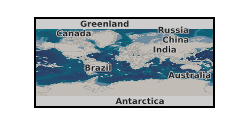Format

## VASP raw outputs

4 record(s)

Type of resources
Topics
Keywords
Contact for the resource
Provided by
Years
Formats
Update frequencies
From 1 - 4 / 4
• # Free energy calculations of noble-gas containing liquid iron and silicate melt (NERC Grant NE/S01134X/1)Free energy calculations of noble-gas containing liquid iron and silicate melts at 50 GPa (3500 K) and 135 GPa (4200 K). The chemical potentials of noble gases can be obtained from these calculations. The Gibbs free energy of a series of iron and silicate melts with different concentrations of He were calculated. Then the chemical potentials of He can be derived from the concentration dependent Gibbs free energies. The chemical potentials of Ne, Ar, Kr and Xe were calculated by using the alchemical free energy method, where one He was converted to Ne, Ar, Kr or Xe gradually.

• # Two-phase modeling of water partitioning between liquid iron and silicate melt (NERC Grant NE/S01134X/1)The two-phase modeling of water between liquid iron and silicate melt at 50 and 135 gigapascals (corresponding to 3500 and 4200 kelvin) was performed by using ab initio molecular dynamics implemented in the Vienna Ab Initio Simulation Package.

• # Water Partitioning between Liquid Iron and Silicate Melt at High Pressure and High Temperature (NERC Grant NE/M015181/1, NE/S01134X/1)The partitioning coefficients of water between iron and silicate melts at 20, 50, 90 and 135 gigapascals (corresponding to 2800, 3500, 3900 and 4200 kelvin) were calculated by using ab initio molecular dynamics and thermodynamic integration techniques. The Gibbs free energy of a series of iron and silicate melts with different concentrations of H2/H2O were calculated. Then the chemical potentials of H2/H2O were derived from the concentration dependent Gibbs free energies at each pressure temperature. The partitioning coefficients can be calculated by equating the chemical potential of H2/H2O in iron and silicate melts. The Weeks-Chandler-Andersen (WCA) system with established thermodynamics was used as the reference.

• # Example Elastic Calculations for ElasT Toolkit (NERC Grant NE/M000125/1)Elastic constants were calculated by using the stress-strain method and density functional theory for crystals of different symmetry. A toolkit was developed to facilitate the input preparation and output processing for elastic calculations using the Vienna Ab Initio Simulation Packge (VASP). The details of the calculation. methods, and the toolkit will be published elsewhere and linked to this deposit. The deposit contains example folders for the monoclinic, orthorhombic, trigonal, tetragonal, hexagonal and cubic lattices. The purpose of this deposit is to provide examples for the toolkit users.